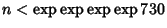## Catalan's Conjecture

8 and 9 (and) are the only consecutive Powers (excluding 0 and 1), i.e., the only solution to Catalan's Diophantine Problem. Solutions to this problem (Catalan's Diophantine Problem) are equivalent to solving the simultaneous Diophantine EquationsThis Conjecture has not yet been proved or refuted, although it has been shown to be decidable in a Finite (but more than astronomical) number of steps. In particular, ifandare Powers, then(Guy 1994, p. 155), which follows from R. Tijdeman's proof that there can be only a Finite number of exceptions should the Conjecture not hold.

Hyyro and Makowski proved that there do not exist three consecutive Powers (Ribenboim 1996), and it is also known that 8 and 9 are the only consecutive Cubic and Square Numbers (in either order).

References

Guy, R. K. Difference of Two Power.'' §D9 in Unsolved Problems in Number Theory, 2nd ed. New York: Springer-Verlag, pp. 155-157, 1994.

Ribenboim, P. Catalan's Conjecture. Boston, MA: Academic Press, 1994.

Ribenboim, P. Catalan's Conjecture.'' Amer. Math. Monthly 103, 529-538, 1996.

Ribenboim, P. Consecutive Powers.'' Expositiones Mathematicae 2, 193-221, 1984.